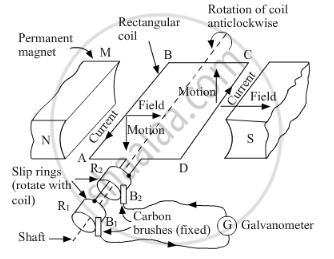Share

# Draw the Labelled Diagram of an A.C. Generator. with the Help of this Diagram, Explain the Construction and Working of an A.C. Generator. - CBSE Class 10 - Science

#### Question

Draw the labelled diagram of an A.C. generator. With the help of this diagram, explain the construction and working of an A.C. generator.

#### Solution

An electric generator (see figure) consists of a rotating rectangular coil ABCD placed between the two poles of a permanent magnet. The two ends of this coil are connected to two rings R1 and R2 . The inner side of these rings are insulated. Two conducting stationary brushes B1 and B2 are kept pressed separately on the rings R1 and R2, respectively. The rings R1 and R2 are internally attached to an axle. The axle may be mechanically rotated from outside to rotate the coil inside the magnetic field. Outer ends of the two brushes are connected to a galvanometer to show the flow of current in the given external circuit.

When the axle is attached to the two rings,  the axle is rotated so that arm AB moves up (and arm CD moves down) in the magnetic field produced by the permanent magnet. Now, coil ABCD is rotated clockwise in an arrangement as shown in the figure. By applying Fleming’s right-hand rule, the induced currents are set up in these arms along the directions of AB and CD. Thus, an induced current flows in the direction ABCD. If there are larger numbers of turns in the coil, the current generated in each turn adds up to give a large current through the coil. This means that the current in the external circuit flows from B2 to B1.
After half rotation, arm CD moves up and arm AB moves down. As a result, the directions of induced currents in both the arms change, giving rise to the net induced current in the direction DCBA. The current in the external circuit now flows from B1 to B2. Thus, after every half rotation, the polarity of currents in respective arms changes. Such a current that changes direction after equal intervals of time is called alternating current (A.C.). This device is called an A.C. generator.Is there an error in this question or solution?

#### APPEARS IN

Lakhmir Singh Solution for Physics for Class 10 (2019 Exam) (2018 to Current)
Chapter 2: Magnetic Effects of Electric Current
Q: 22 | Page no. 103

#### Video TutorialsVIEW ALL 

Solution Draw the Labelled Diagram of an A.C. Generator. with the Help of this Diagram, Explain the Construction and Working of an A.C. Generator. Concept: Electric Generator - AC.
S